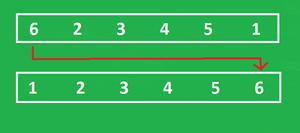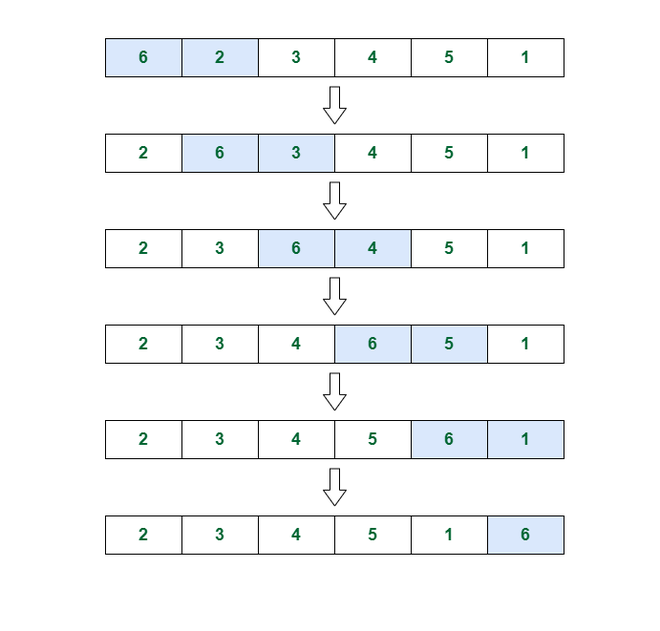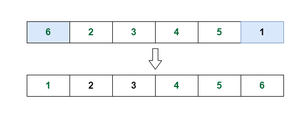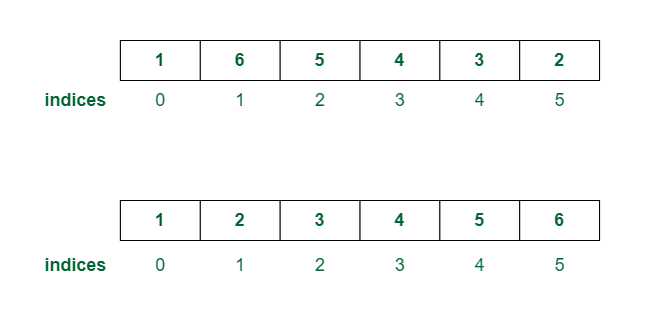GFG App
Open AppBrowser
Continue

# Maximize X such that Array can be sorted by swapping elements X distance apart

Given an input arr[] of length N, Which contains integers from 1 to N in unsorted order. You can apply only one type of operation on arr[] elements’:

• Choose an index i and an integer X and swap Ai with an element that is X distance apart and both the indices are within the boundary of the array.

Then the task is to find the maximum value of X by which all the elements should be at their respective place of sorted order.

Examples:

Input: N = 6, arr[] = {6, 2, 3, 4, 5, 1}
Output: 5
Explanation: arr[] can be sorted by exchanging elements at first (A1 = 6)
and last(A6 = 1) index of arr[]. Here value of X is 5 and also follows the rule of given operation.
It can be verified that there is no max value rather than 5 of  by which arr[] can be sorted.

Input: N = 6, arr[] = {3, 2, 1, 6, 5, 4}
Output: 2
Explanation: Element at 1st and 3rd  index can be swapped
to form the arr[] as = {1, 2, 3, 6, 5, 4} and then 4th  and 6th
element can be exchanged with each other to make arr[] sorted: {1, 2, 3, 4, 5, 6}.
For first operation value of X used : 2
For second operation value of X used : 2
Then, Maximum value of X among all operations by which
we can place all elements to their respective sorted position: 2

Approach: Implement the below idea to solve the problem

Calculate the absolute difference between index of sorted and unsorted position of each element and take GCD of all absolute differences to find the maximum value of X.

Proof of the approach:

The approach contains uses two things:

• Absolute differences between the index of the actual(unsorted) and sorted position of each element.
• GCD of all differences.

Let’s understand them one by one:

• The idea behind absolute difference:Sorting arr[] by exchanging 6 with 1

In the above image, the first array is unsorted and the second one is in a sorted format. We can clearly see that arr[] can be sorted by swapping elements 1 and 6.

Now, Just think about the element: 6, which is at the first index of unsorted arr[]. Forget about rest of the elements for now. Observe that we have 2 possible values of X = (1, 5)  by which we can reach 6 to its sorted position.

When X = 1:
Initially unsorted arr[] = {6, 2, 3, 4, 5, 1}

On swapping 6 with next Xth element  = (2) in arr[] ={6, 2, 3, 4, 5, 1}, Then arr[] will be  = {2, 6, 3, 4, 5, 1}
On swapping 6 with next Xth  element = (3) in arr[] = {2, 6, 3, 4, 5, 1}, Then arr[] will be  = {2, 3, 6, 4, 5, 1}
On swapping 6 with next Xth element = (4) in arr[] = {2, 3, 6, 4, 5, 1}, Then arr[] will be= {2, 3, 4, 6, 5, 1}
On swapping 6 with next Xth element = (5) in arr[] = {2, 3, 4, 6, 5, 1}, Then arr[] will be = {2, 3, 4, 5, 6, 1}
On swapping 6 with next Xth element = (1) in arr[] = {2, 3, 4, 5, 6, 1}, Then arr[] will be = {2, 3, 4, 5, 1, 6}Illustration of the process when X = 1

Important Note: All elements are not in their respective sorted position, As we were talking about the only element: 6, So above operations were just for element 6.

When X = 5:

Initially unsorted arr[] = {6, 2, 3, 4, 5, 1}

On exchanging 6 with next Xth element  = (1) in arr[] ={6, 2, 3, 4, 5, 1}, Then arr[] will be  = {1, 2, 3, 4, 5, 6}
We successfully put 6 to its respective sorted position in both of the cases of X. So now the question is from where we got 1 and 5 for X?

The explanation for possible values of X:

Value of index, When element 6 was present in unsorted arr[] = 1(1 based indexing)
Value of index, When element 6 was present in sorted arr[] = 6(1 based indexing)
Absolute difference between sorted and unsorted index = | 6 – 1 | = 5.Illustration for the case of x = 5

To cover the distance of 5 indices, We must choose the value of X in such a way that the difference should be completely divisible by X. Which conclude that X should be a factor of absolute difference

Formally, All possible values of X for a single element are = All factors of absolute difference. It can be verified that 6 can’t be placed to its respective sorted position by choosing X = {2, 3, 4}, Because none of them are factors of absolute difference=5. Therefore, for the above-discussed case, we have two possible values of X, in Which 5 is the Maximum.

As we know that any number is a factor in itself. Therefore we can conclude the rule:-

Suppose absolute difference = K. All possible values of X  = factors(K) = {A, B, . . . . ., K}.It can be also verified that K will be the maximum among all possible factors. Formally:-

Max((factors(K)) = K = Maximum possible value of X for an element.

Therefore, maximum possible value of X(Not for the final answer of the problem, just for a single element of arr[], which is 6 in this case) will be equal to the absolute difference between the index of the sorted and unsorted position of an element. Hence, the First thing of approach proves successful.

• The idea behind calculating GCD of all absolute differences:Unsorted and sorted array with indices

For the case in the above image:-

As we can see that 6, 5, and 2 are not in their respective sorted position, Therefore:-

• Absolute difference of indices for element 6 = |2 – 6| = 4
• Max value of X for element 6 = Max(factors(4)) = Max(1, 2, 4) =  4.
• Absolute difference of indices for element 5 = |3 – 5| = 2
• Max value of X for element 5 = Max(factors(2)) = Max(1, 2) = 2.
• Absolute difference of indices for element 2 = |6 – 2| = 4
• Max value of X for element 2 = Max(factors(4)) = Max(1, 2, 4) = 4.

These are the maximum values of X for each element, Which are not initially present in its sorted position. Now we have to find a  Maximum value of X for all unsorted elements by which they should be at their sorted place(Formally, said to make arr[] sorted) under finite number of operations. For X should be chosen in such a way that it covers all the absolute differences i.e., divisor of all the absolute differences as well as the greatest possible. This can be only done when X is the Greatest Common Divisor(GCD) of all absolute differences.

Follow the steps mentioned below to implement the idea:

• Traverse through the array from i = 0 to N-1:
• Find the absolute difference of each element from their sorted position by finding the difference between (i+1) and the element [because the array contains values from 1 to N and in their sorted order i+1 will be in ith index.
• Find the GCD of all the differences and return that as the required answer.

Below is the implementation for the above approach:

## C++

 `// c++ implementation` `#include ` `using` `namespace` `std;`   `// Euclidean algorithm to return` `// GCD of two numbers` `int` `GCD(``int` `a, ``int` `b)` `{` `  ``return` `b == 0 ? a : GCD(b, a % b);` `}`     `int` `MAX_X(``int` `n, ``int` `arr[])` `{`   `  ``// Variable to Store max value of X` `  ``int` `X = 0;` `  ``for` `(``int` `i = 0; i < n; i++)` `  ``{`   `    ``// Calculating GCD of` `    ``// absolute differences` `    ``X = GCD(``abs``((i + 1) - arr[i]), X);` `  ``}`   `  ``// Returning Max value of X` `  ``// from the function.` `  ``return` `X;` `}`   `int` `main()` `{`   `  ``// Input value of N` `  ``int` `n = 6;`   `  ``// Input arr[]` `  ``int` `arr[] = {6, 2, 3, 4, 5, 1};`   `  ``// Printing Maximum value of X by` `  ``// calling MAX_X() function` `  ``cout<

## Java

 `import` `java.util.*;` `public` `class` `GFG {`   `    ``// Driver function` `    ``public` `static` `void` `main(String[] args)` `    ``{` `        ``// Input value of N` `        ``int` `n = ``6``;`   `        ``// Input arr[]` `        ``int``[] arr = { ``6``, ``2``, ``3``, ``4``, ``5``, ``1` `};`   `        ``// Printing Maximum value of X by` `        ``// calling MAX_X() function` `        ``System.out.println(MAX_X(n, arr));` `    ``}`   `    ``// Function to find maximum value of X` `    ``static` `int` `MAX_X(``int` `n, ``int``[] arr)` `    ``{`   `        ``// Variable to Store max value of X` `        ``int` `X = ``0``;` `        ``for` `(``int` `i = ``0``; i < n; i++) {`   `            ``// Calculating GCD of` `            ``// absolute differences` `            ``X = GCD(Math.abs((i + ``1``) - arr[i]), X);` `        ``}`   `        ``// Returning Max value of X` `        ``// from the function.` `        ``return` `X;` `    ``}`   `    ``// Euclidean algorithm to return` `    ``// GCD of two numbers` `    ``static` `int` `GCD(``int` `a, ``int` `b)` `    ``{` `        ``return` `b == ``0` `? a : GCD(b, a % b);` `    ``}` `}`

## Python3

 `import` `math` `class` `GFG :` `  `  `    ``# Driver function` `    ``@staticmethod` `    ``def` `main( args) :` `      `  `        ``# Input value of N` `        ``n ``=` `6` `        `  `        ``# Input arr[]` `        ``arr ``=` `[``6``, ``2``, ``3``, ``4``, ``5``, ``1``]` `        `  `        ``# Printing Maximum value of X by` `        ``# calling MAX_X() function` `        ``print``(GFG.MAX_X(n, arr))` `        `  `    ``# Function to find maximum value of X` `    ``@staticmethod` `    ``def`  `MAX_X( n,  arr) :` `      `  `        ``# Variable to Store max value of X` `        ``X ``=` `0` `        ``i ``=` `0` `        ``while` `(i < n) :` `          `  `            ``# Calculating GCD of` `            ``# absolute differences` `            ``X ``=` `GFG.GCD(``abs``((i ``+` `1``) ``-` `arr[i]), X)` `            ``i ``+``=` `1` `            `  `        ``# Returning Max value of X` `        ``# from the function.` `        ``return` `X` `      `  `    ``# Euclidean algorithm to return` `    ``# GCD of two numbers` `    ``@staticmethod` `    ``def`  `GCD( a,  b) :` `        ``return` `a ``if` `b ``=``=` `0` `else` `GFG.GCD(b, a ``%` `b)` `    `  `if` `__name__``=``=``"__main__"``:` `    ``GFG.main([])` `    `  `    ``# This code is contributed by aadityaburujwale.`

## C#

 `// Include namespace system` `using` `System;`     `public` `class` `GFG` `{` `    ``// Driver function` `    ``public` `static` `void` `Main(String[] args)` `    ``{` `        ``// Input value of N` `        ``var` `n = 6;` `      `  `        ``// Input arr[]` `        ``int``[] arr = {6, 2, 3, 4, 5, 1};` `      `  `        ``// Printing Maximum value of X by` `        ``// calling MAX_X() function` `        ``Console.WriteLine(GFG.MAX_X(n, arr));` `    ``}` `    ``// Function to find maximum value of X` `    ``public` `static` `int` `MAX_X(``int` `n, ``int``[] arr)` `    ``{` `      `  `        ``// Variable to Store max value of X` `        ``var` `X = 0;` `        ``for` `(``int` `i = 0; i < n; i++)` `        ``{` `          `  `            ``// Calculating GCD of` `            ``// absolute differences` `            ``X = GFG.GCD(Math.Abs((i + 1) - arr[i]), X);` `        ``}` `      `  `        ``// Returning Max value of X` `        ``// from the function.` `        ``return` `X;` `    ``}` `  `  `    ``// Euclidean algorithm to return` `    ``// GCD of two numbers` `    ``public` `static` `int` `GCD(``int` `a, ``int` `b)` `    ``{` `        ``return` `b == 0 ? a : GFG.GCD(b, a % b);` `    ``}` `}`   `// This code is contributed by aadityaburujwale.`

## Javascript

 `// JavaScript implementation`   `// Euclidean algorithm to return` `// GCD of two numbers` `const GCD = (a, b) => {` `    ``return` `b == 0 ? a : GCD(b, a % b);` `}`   `const MAX_X = (n, arr) => {`   `    ``// Variable to Store max value of X` `    ``let X = 0;` `    ``for` `(let i = 0; i < n; i++) {`   `        ``// Calculating GCD of` `        ``// absolute differences` `        ``X = GCD(Math.abs((i + 1) - arr[i]), X);` `    ``}`   `    ``// Returning Max value of X` `    ``// from the function.` `    ``return` `X;` `}`   `// Input value of N` `let n = 6;`   `// Input arr[]` `let arr = [6, 2, 3, 4, 5, 1];`   `// Printing Maximum value of X by` `// calling MAX_X() function` `console.log(MAX_X(n, arr));`   `// This code is contributed by rakeshsahni`

Output

`5`

Time complexity: O(N * log(Max)), Where Max is maximum element present in arr[].
Auxiliary Space: O(1) is, As no extra space is used.

My Personal Notes arrow_drop_up